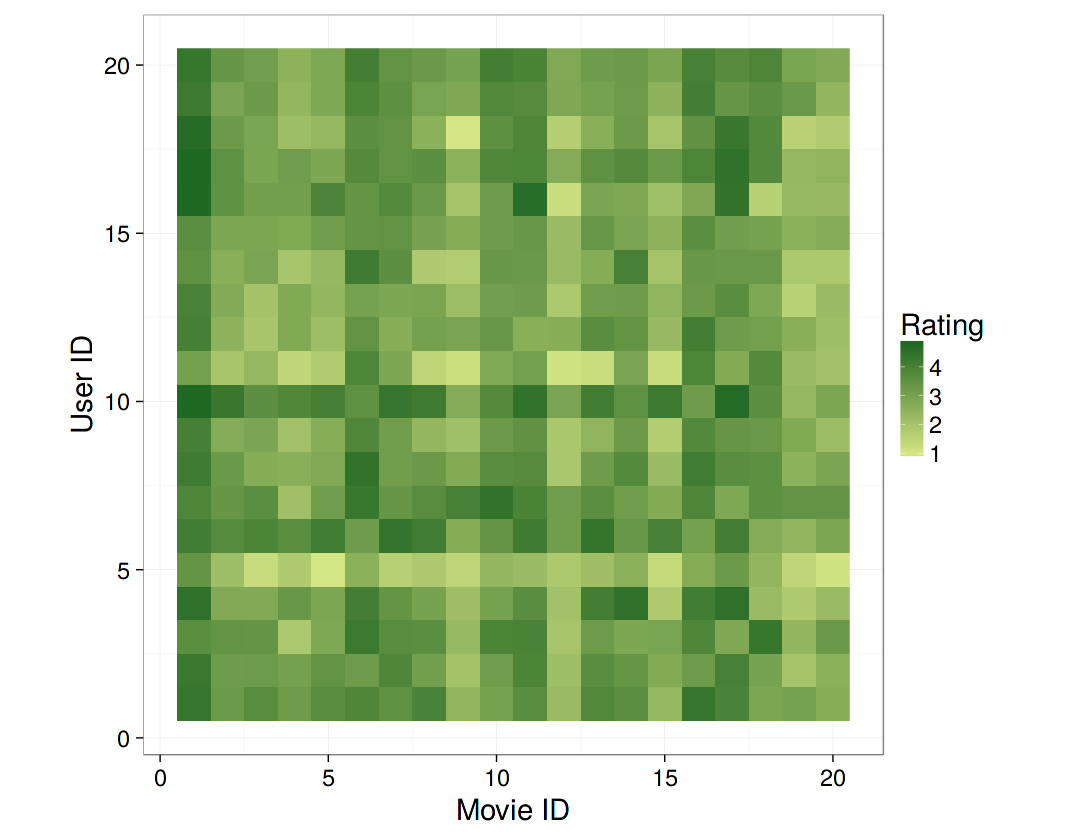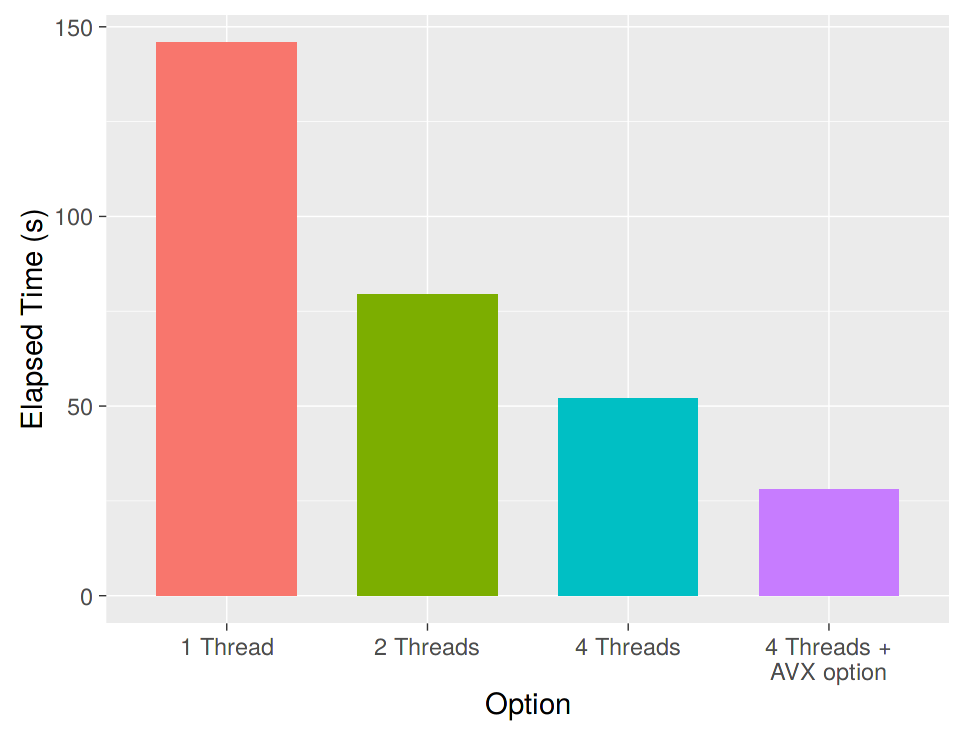# A Quick View of Recommender System

The main task of recommender system is to predict unknown entries in the rating matrix based on observed values, as is shown in the table below:Each cell with number in it is the rating given by some user on a specific item, while those marked with question marks are unknown ratings that need to be predicted. In some other literatures, this problem may be named collaborative filtering, matrix completion, matrix recovery, etc.

A popular technique to solve the recommender system problem is the matrix factorization method. The idea is to approximate the whole rating matrix $R_{m\times n}$ by the product of two matrices of lower dimensions, $P_{k\times m}$ and $Q_{k\times n}$, such that

$$R\approx P^\prime Q$$

Let $p_u$ be the $u$-th column of $P$, and $q_v$ be the $v$-th column of $Q$, then the rating given by user $u$ on item $v$ would be predicted as $p^\prime_u q_v$.

A typical solution for $P$ and $Q$ is given by the following optimization problem [1; 2]:

$$\min_{P,Q} \sum_{(u,v)\in R} \left[f(p_u,q_v;r_{u,v})+\mu_P||p_u||_1+\mu_Q||q_v||_1+\frac{\lambda_P}{2} ||p_u||_2^2+\frac{\lambda_Q}{2} ||q_v||_2^2\right]$$

where $(u,v)$ are locations of observed entries in $R$, $r_{u,v}$ is the observed rating, $f$ is the loss function, and $\mu_P,\mu_Q,\lambda_P,\lambda_Q$ are penalty parameters to avoid overfitting.

The process of solving the matrices $P$ and $Q$ is called model training, and the selection of penalty parameters is parameter tuning. After obtaining $P$ and $Q$, we can then do the prediction of $\hat{R}_{u,v}=p^\prime_u q_v$.

# LIBMF and recosystem

LIBMF is an open source C++ library for recommender system using parallel matrix factorization, developed by Dr. Chih-Jen Lin and his research group. 

LIBMF is a parallelized library, meaning that users can take advantage of multi-core CPUs to speed up the computation. It also utilizes some advanced CPU features to further improve the performance.

recosystem (Github) is an R wrapper of the LIBMF library that inherits most of its features. Additionally, this package provides a number of user-friendly R functions to simplify data processing and model building. Also, unlike most other R packages for statistical modeling that store the whole dataset and model object in memory, LIBMF (and hence recosystem) can significantly reduce memory use, for instance the constructed model that contains information for prediction can be stored in the hard disk, and output result can also be directly written into a file rather than be kept in memory.

# Overview of recosystem

The usage of recosystem is quite simple, mainly consisting of the following steps:

1. Create a model object (a Reference Class object in R) by calling Reco().
2. Specify the data source, either from a data file or from R objects in memory.
3. Train the model by calling the $train() method. A number of parameters can be set inside the function. 4. (Optionally) Call the $tune() method to select best tuning parameters along a set of candidate values, in order to achieve better model performance.
5. (Optionally) Export the model via $output(), i.e. write the factorization matrices$P$and$Q$into files or return them as R objects. 6. Use the $predict() method to compute predicted values.

More details are covered in the package vignette and the help pages ?recosystem::Reco, ?recosystem::data_source, ?recosystem::train, ?recosystem::tune, ?recosystem::output, and ?recosystem::predict.

In the next section we will demonstrate how to use recosystem to analyze a real movie recommendation data set.

# MovieLens Data

The MovieLens website collected many movie rating data for research use.  In this article we download the MovieLens 1M Dataset from grouplens, which contains 1 million ratings from 6000 users and 4000 movies.

The rating data file, ratings.dat, looks like below:

1::1193::5::978300760
1::661::3::978302109
1::914::3::978301968
1::3408::4::978300275
1::2355::5::978824291
...


Each line has the format UserID::MovieID::Rating::Timestamp, for example the first line says that User #1 gave Movie #1193 a rating of 5 at certain time point.

In recosystem, we will not use the time information, and the required data format is UserID MovieID Rating, i.e., the columns are space-separated, and columns after Rating will be ignored. Therefore, we first transform the data file into a format that is supported by recosystem. On Unix-like OS’s, we can use the sed command to replace :: by a space:

sed -e 's/::/ /g' ratings.dat > ratings2.dat


Then we can start to train a recommender, as the following code shows:

library(recosystem)                                             # 1
r = Reco()                                                      # 2
train_set = data_file("ratings2.dat",  index1 = TRUE)           # 3
r$train(train_set, opts = list(dim = 20, # 4 costp_l1 = 0, costp_l2 = 0.01, # 5 costq_l1 = 0, costq_l2 = 0.01, # 6 niter = 10, # 7 nthread = 4)) # 8  In the code above, line 2 creates a model object such that the training function $train() can be called from it. Line 3 specifies the data source – a data file on hard disk. Since in our data user ID and movie ID start from 1 rather than 0, we use the index1 = TRUE options in the function.

The data can also be read from memory, if the UserID, MovieID and Rating columns are stored as R vectors. Below shows an alternative way to provide the training set.

dat = read.table("ratings2.dat", sep = " ", header = FALSE,
colClasses = c(rep("integer", 3), "NULL"))
train_set = data_memory(user_index = dat[, 1],
item_index = dat[, 2],
rating = dat[, 3], index1 = TRUE)


Line 4 to line 6 set the relevant model parameters: $k, \mu_P,\mu_Q,\lambda_P$, and $\lambda_Q$, and Line 7 gives the number of iterations. Finally as I have mentioned previously, LIBMF is a parallelized library, so users can specify the number of threads that will be working simultaneously via the nthread parameter. However, when nthread > 1, the training result is NOT guaranteed to be reproducible, even if a random seed is set.

Now everything looks good, except one inadequacy: the setting of tuning parameters is ad-hoc, which may make the model sub-optimal. To tune these parameters, we can call the $tune() function to test a set of candidate values and use cross validation to evaluate their performance. Below shows this process: opts_tune = r$tune(train_set,                                   # 9
opts = list(dim      = c(10, 20, 30),        # 10
costp_l2 = c(0.01, 0.1),         # 11
costq_l2 = c(0.01, 0.1),         # 12
costp_l1 = 0,                    # 13
costq_l1 = 0,                    # 14
lrate    = c(0.01, 0.1),         # 15
niter    = 10,                   # 17
verbose  = TRUE))                # 18
r$train(train_set, opts = c(opts_tune$min,                      # 19
niter = 100, nthread = 4))          # 20


The options in line 9 to line 15 are tuning parameters. The tuning function will evaluate each combination of them and calculate the associated cross-validated RMSE. The parameter set with the smallest RMSE will be contained in the returned value, which can then be passed to $train() (Line 19-20). Finally, we can use the model object to do predictions. The code below shows how to predict ratings given by the first 20 users on the first 20 movies. user = 1:20 movie = 1:20 pred = expand.grid(user = user, movie = movie) test_set = data_memory(pred$user, pred$movie, index1 = TRUE) pred$rating = r\$predict(test_set, out_memory())

library(ggplot2)
ggplot(pred, aes(x = movie, y = user, fill = rating)) +
geom_raster() +
scale_fill_gradient("Rating", low = "#d6e685", high = "#1e6823") +
xlab("Movie ID") + ylab("User ID") +
coord_fixed() +
theme_bw(base_size = 22)# Performance

To make the best use of recosystem, the parallel computing option nthread should be used in the training and tuning step. Also, LIBMF and recosystem can make use of some advanced CPU features to speed-up computation, if you compile the package from source and turn on some compiler options.

To build recosystem, one needs a C++ compiler that supports the C++11 standard. Then you can edit src/Makevars (src/Makevars.win for Windows system) according to the following guideline:

• The default Makevars provides generic options that should apply to most CPUs.
• If your CPU supports SSE3 (a list of supported CPUs), add
PKG_CPPFLAGS += -DUSESSE
PKG_CXXFLAGS += -msse3

PKG_CPPFLAGS += -DUSEAVX
PKG_CXXFLAGS += -mavx


After editing the Makevars file, run R CMD INSTALL recosystem to install recosystem.

The plot below shows the effect of parallel computing and the compiler option on the performance of computation. The y axis is the elapsed time of the model tuning procedure in the previous example.Chin, Wei-Sheng, Yong Zhuang, Yu-Chin Juan, and Chih-Jen Lin. 2015a. A Fast Parallel Stochastic Gradient Method for Matrix Factorization in Shared Memory Systems. ACM TIST.

 Chin, Wei-Sheng, Yong Zhuang, Yu-Chin Juan, and Chih-Jen Lin. 2015b. A Learning-Rate Schedule for Stochastic Gradient Methods to Matrix Factorization. ACM TIST.

 Lin, Chih-Jen, Yu-Chin Juan, Yong Zhuang, and Wei-Sheng Chin. 2015. LIBMF: A Matrix-Factorization Library for Recommender Systems.

 F. Maxwell Harper and Joseph A. Konstan. 2015. The MovieLens Datasets: History and Context. ACM Transactions on Interactive Intelligent Systems (TiiS) 5, 4, Article 19 (December 2015), 19 pages.